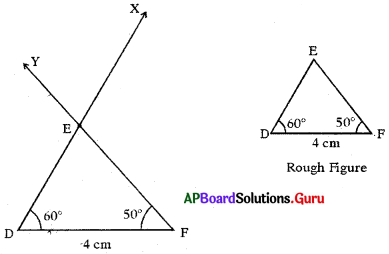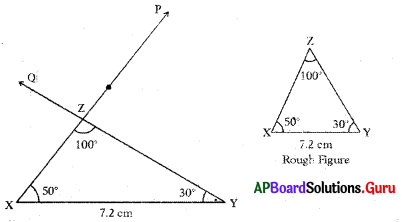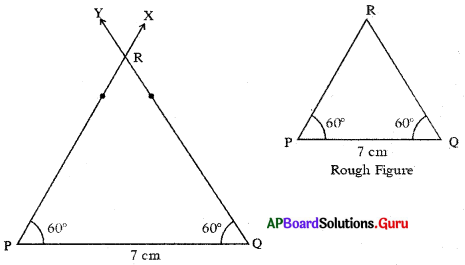SCERT AP 7th Class Maths Solutions Pdf Chapter 10 Construction of Triangles Ex 10.3 Textbook Exercise Questions and Answers.

## AP State Syllabus 7th Class Maths Solutions 10th Lesson Construction of Triangles Ex 10.3

Question 1.
Construct ∆DEF with measurements ∠D = 60°, ∠F = 50° and DF = 4 cm.
Given measurements of ∆DEF are ∠D = 60°, ∠F = 50° and DF =. 4 cm.Steps of Construction :

1. Draw a rough sketch of triangle and label it with given measurements.
2. Draw a line segment with DF = 4 cm.
3. Draw a ray DX such that ∠FDX = 60°.
4. Draw another ray FY such that ∠DFY = 50° which intersects the previous ray at E. Thus, required ∆DEF is constructed with the given measurements.Question 2.
Construct the triangle with the measurements XY = 7.2 cm, ∠Y = 30° and ∠Z = 100°.
Given measurements of ∆XYZ are XY = 7.2 cm, ∠Y = 30° and ∠Z = 100°.
We know stun of angles of triangle is 180°
So, ∠X + ∠Y + ∠Z = 180°
⇒ ∠X + 30° + 100° = 180°
⇒ ∠X= 180°-130°
∴ ∠X = 50°Steps of Construction:

1. Draw a rough sketch of triangle and label it with given measurements.
2. Draw a line segment with XY = 7.2 cm.
3. Draw a ray $$\overrightarrow{\mathrm{XP}}$$ such that ∠YXP = 50°
4. Draw another ray $$\overrightarrow{\mathrm{YQ}}$$ such that ∠XYQ = 30° which intersects the previous ray at Z. Thus, required ∆XYZ is constructed with the given measurements.

Question 3.
Construct ∆PQR with the measurements ∠P = ∠Q = 60° and PQ = 7 cm.